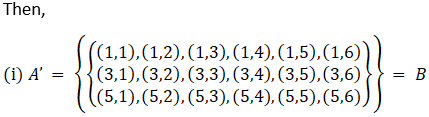Two dice are thrown. The events A, B and C are as follows:

A: getting an even number on the first die.

B: getting an odd number on the first die.

C: getting the sum of the numbers on the dice ≤ 5.

Describe the events

(i) AI

(ii) not B

(iii) A or B

(iv) A and B

(v) A but not C

(vi) B or C

(vii) B and C

(viii) A ∩ BI ∩ CI

Asked by Abhisek | 1 year ago |  136

##### Solution :-

Let us assume that 1, 2, 3, 4, 5 and 6 are the possible out comes when the die is thrown.

In the question is given that pair of die is thrown, so sample space will be,Accordingly,Answered by Pragya Singh | 1 year ago

### Related Questions

#### One number is chosen from numbers 1 to 100. Find the probability that it is divisible by 4 or 6?

One number is chosen from numbers 1 to 100. Find the probability that it is divisible by 4 or 6?

#### The probability that a student will pass the final examination in both English and Hindi is 0.5

The probability that a student will pass the final examination in both English and Hindi is 0.5 and the probability of passing neither is 0.1. If the probability of passing the English examination is 0.75. What is the probability of passing the Hindi examination?

#### A card is drawn from a deck of 52 cards. Find the probability of getting an ace or a spade card.

A card is drawn from a deck of 52 cards. Find the probability of getting an ace or a spade card.